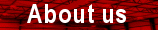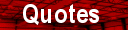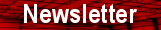HeatersspacerSearch this site: Using :  All Words  Any Words PhraseW. Tombling Ltd.

Wembley House
Dozens Bank
West Pinchbeck
Spalding
Lincolnshire
PE11 3ND
U.K.

Telephone
+44 (0) 1775 640 049

Facsimile
+44 (0) 1775 640 050

Email
mail@tombling.com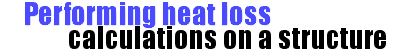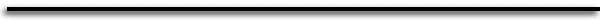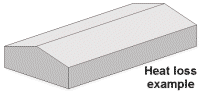Dimensions (metres) Length Width Eave  height Ridge height 30 10 2 3 'U' values (W/m2deg.C) Walls (brick 228mm) Roof   (corrugated asbestos) Floor  (concrete) 2.6 5.7 0.7 Design temp. (deg.C) Min. ambient (outside) temp. Required inside temp. - 5 20'U' values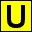'U' values for commonly used building materials.
Normal exposure conditions.
(W/m2deg.C).

 Basic materials Glass 5.7 Corrugated Asbestos 5.7 Sheet Asbestos 6.5 Wood 25mm 5.0 Brick (unplastered) 228mm 2.6 Concrete 100mm solid 4.0 Earth floor 1.9 Concrete floor 0.7 Windows Single glazed wood 4.3 Single glazed metal 5.6 Double glazed wood 2.5 Double glazed metal 3.2 Composite materials Insulation block 140mm rendered both sides 1.1 Corrugated double cladding with 25mm glassfibre over polythene vapour barrier 1.4 70mm extruded polystyrene  bonded to 6mm fibre cement each side 0.43 40mm glassfibre over polyethylene vapour barrier and 3mm fibre cement 0.5 60mm glassfibre over polyethylene vapour barrier and 3mm fibre cement 0.4 140mm thick concrete block rendered outside, lined inside with timber battens, 40mm extruded polystyrene and flat fibre cement sheet 0.44 Plywood exterior cladding, 40mm extruded polystyrene, fibre lining 0.46How to calculate the heat loss for a structure

To calculate the size of heater(s) required to heat a structure, we need to know:

• The temperature to be maintained within the structure.

• The lowest ambient (outside temperature) which can be expected for the area.

• The direct heat loss from the overall surface area of the structure.

• Heat loss through natural or mechanical ventilation.

The difference between the ambient and internal temperatures gives the temperature lift required.

Temperature lift = Internal temp. - ambient temp.

The heat loss for a structure is calculated by taking each surface in turn, calculating its overall area and multiplying by its thermal transmittance co-efficient or ‘U - value’ (see table)

Heat loss through surface = width(m) x length(m) x U value

Note the area of windows and doors should be calculated and deducted from the area of the surface they are in, and their heat loss should be calculated separately.

The total surface heat loss for the structure is the sum of all surface heat losses.

Total surface heat loss = loss for walls + loss for roof +   loss for floor + loss for windows + loss for doors

An allowance should be made for heat loss through ventilation, which also includes leaks of air through badly fitting doors, windows, damage to the structures surface etc. Putting a value on this can be very difficult, figures range from 20% to 66% depending on the type and condition of the structure.

Total heat loss = total surface heat loss x allowance for heat loss through ventilation

Finally to calculate the heater size needed, the total heat loss is multiplied by the temperature lift.

Heater size required = total heat loss x temperature lift

Example heat loss calculation (see diagram)

Temperature lift = 20 - (-5) = 25 deg. C

Area of roof = 2 x 5.09 x 30 = 305.4 m2
Area of walls = (2x2x30) + 10(2+3) = 180 m2
Area of floor = 30 x 10 = 300 m2

Heat loss through roof = 305.4 x 5.7 = 1740 W
Heat loss through walls = 180 x 2.6 = 468 W
Heat loss through floor = 300 x 0.7 = 210 W

Total surface heat loss = 2418 W

Assuming 20% for heat loss through ventilation
Total heat loss = 1.2 x 2418 = 2901 W

Heater size required = total heat loss x temperature lift                = 2901 x 25 = 72525 W

In this example 4 - 20kW Activair Ace electric fan heaters , 5 - 15kW Activair portable heaters or 4- 21kW Activair wall mounted electric heaters are needed.

Calculating heater running costs per hour

Calculating the running cost per hour for an electric heater is straightforward. Electricity is sold by the unit (kWh), multiply this by the heater size in kW.

Running cost per hour = heater size (kW) x unit cost of electricity (kWh)

Estimating annual heater running costs

Annual running costs will be greatly affected by physical and geographical location and prevailing weather conditions from year to year. To calculate approximate running costs heating degree day tables are available for most areas, based on average ambient temperatures over a number of years. (contact your local meteorological office or search the internet for ‘heating degree day table’) Consult the heating degree day table to find the annual figure for the temperature the structure is to be maintained at.

Estimated annual energy requirement (kWh)= total heat loss x 86400 x figure from degree table/3600000

Finally to calculate the annual running cost multiply the estimated annual energy requirement by the unit cost of your electricity.

Annual running cost = annual energy requirement x unit cost of electricity

Disclaimer. The above example is greatly simplified. U values of building materials vary and are affected by numerous conditions including the position of the structure, i.e. sheltered, normal or exposed. The above should be considered as an approximate method of calculating heater size and running costs. W. Tombling Ltd. accepts no liability or claim arising from use of this information.